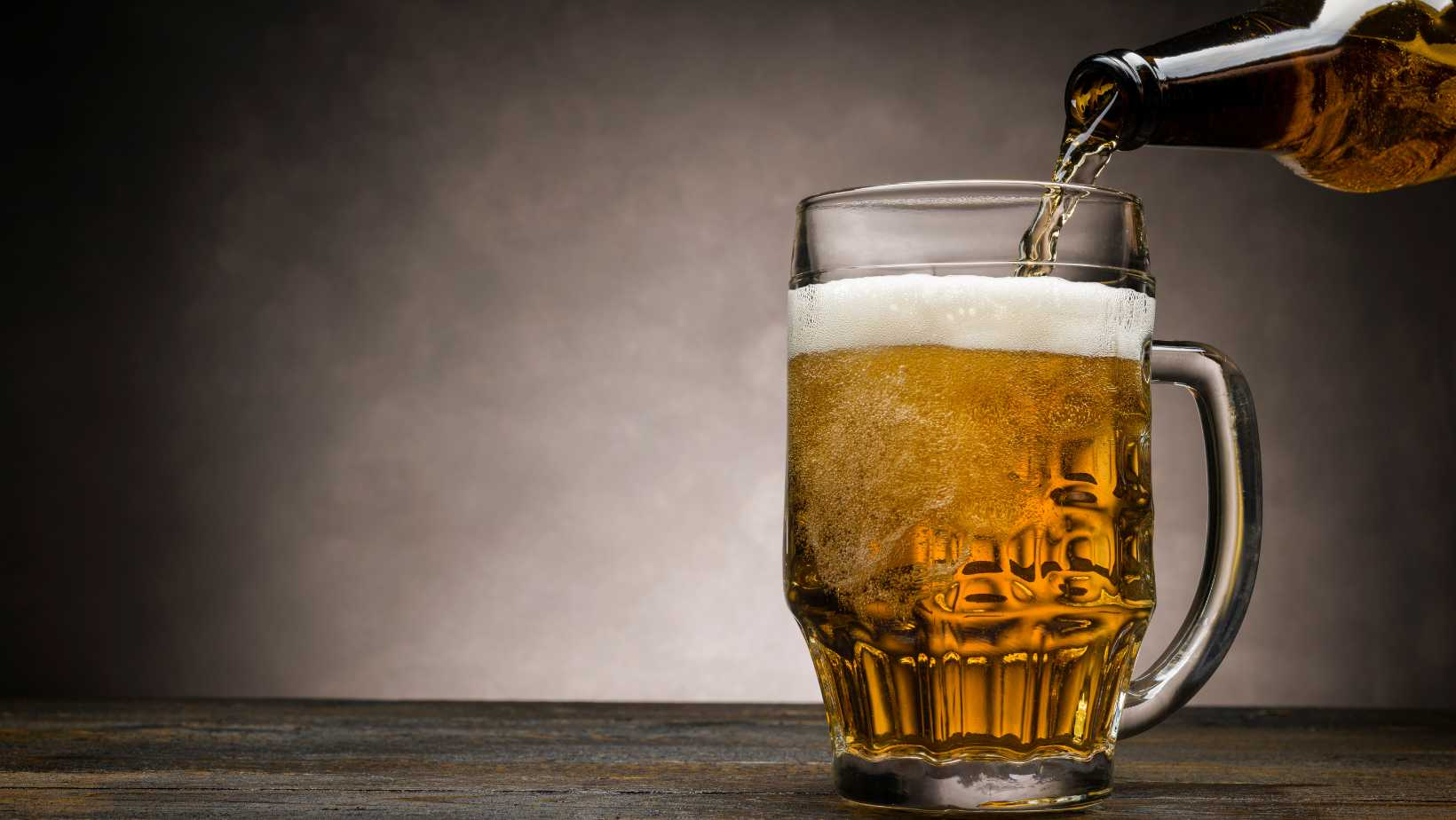# How Many mL in a Pint? A Quick Tip to ConversionWondering how many milliliters are in a pint? Well, let me clear that up for you. In the United States, there are 473.18 milliliters in one pint. So, if you’re measuring liquids or ingredients and need to convert from pints to milliliters, simply multiply the number of pints by 473.18.

Understanding the conversion between pints and milliliters can be helpful when following recipes or working with different measurement systems. It’s important to keep in mind that this conversion applies specifically to US customary units of measure.

Next time you come across a recipe calling for a certain amount of liquid measured in pints, remember that it’s equivalent to approximately 473.18 milliliters. Having this knowledge at your fingertips will make it easier to navigate through various cooking and baking adventures.

Whether you’re a seasoned chef or just starting out in the kitchen, knowing how many milliliters are in a pint can save you time and ensure accurate measurements for delicious results.

## How Many mL in a Pint?

When it comes to converting measurements, it’s essential to know how many milliliters are in a pint. Whether you’re working in the kitchen or dealing with fluid quantities, understanding this conversion can be incredibly useful. In this section, we’ll explore the relationship between pints and milliliters and how to convert between them effortlessly.

### The Conversion: Pints to Milliliters

To determine the number of milliliters in a pint, we need to consider that one pint is equal to 473.176 milliliters (ml). This precise conversion allows for accurate calculations when dealing with fluid volumes. For example:

• If you have two pints of milk and want to know how many milliliters that would be, simply multiply 2 by 473.176 ml.
* Result: 2 pints x 473.176 ml/pint = 946.352 ml

It’s important to remember that this conversion factor remains consistent regardless of the substance being measured.

### Different Measurements: Milliliters and Pints

While both milliliters and pints are used as units of liquid measurement, they belong to different systems. Milliliters are part of the metric system, widely adopted worldwide for its simplicity and precision. On the other hand, pints are commonly used in countries like the United States and the United Kingdom as part of their customary measurement system.

The disparity between these two systems can lead to confusion when trying to convert measurements accurately or compare quantities across different regions. However, knowing how many milliliters are equivalent to a pint enables seamless conversions between metric and imperial units.

### Converting Fluids: Pints to Milliliters

Converting from pints to milliliters is straightforward once you understand the conversion factor mentioned earlier (1 pint = 473.176 ml). Here’s a simple step-by-step process to guide you:

1. Identify the number of pints you want to convert.
2. Multiply the number of pints by 473.176 ml/pint.
3. Round the result to the desired level of precision, if necessary.

For example, let’s say you have 3 pints of water and need to convert it into milliliters:

* Calculation: 3 pints x 473.176 ml/pint = 1419.528 ml

* Rounded Result: Approximately 1419.53 ml

By following this conversion process, you can accurately determine fluid volumes in milliliters based on a given quantity in pints.

## Converting Pints to Milliliters

When it comes to converting pints to milliliters, it’s important to have a clear understanding of the conversion factor between these two units of measurement. So, let’s dive right in and explore how many milliliters are there in a pint.

A pint is commonly used as a unit of volume in both the US customary system and the British imperial system. In the US, a pint is equal to 16 fluid ounces or approximately 473 milliliters. On the other hand, in the British imperial system, a pint is equivalent to 20 fluid ounces or about 568 milliliters.

To make things easier, here’s a quick reference table for you:

 Pint (US) Milliliters 1 473 2 946 3 1,419 … …

Please note that these values are approximate conversions and may vary slightly depending on rounding methods or regional variations.

Now that we have established the conversion factor for pints to milliliters, you can easily calculate any desired value by multiplying the number of pints by either 473 (for US pints) or 568 (for British imperial pints).

For example:

• To convert 4 pints into milliliters:
• For US pints:
• Number of pints: 4
• Conversion factor: 473 ml/pint
• Calculation: 4 x 473 = 1892 ml
• For British imperial pints:
• Number of pints: 4
• Conversion factor: 568 ml/pint
• Calculation: 4 x 568 = 2272 ml

So, whether you’re working with US pints or British imperial pints, now you know how to convert them into milliliters accurately.

I hope this information helps answer your question on how many milliliters are there in a pint.

Amanda is the proud owner and head cook of her very own restaurant. She loves nothing more than experimenting with new recipes in the kitchen, and her food is always a big hit with customers. Amanda takes great pride in her work, and she always puts her heart into everything she does. She's a hard-working woman who has made it on her own, and she's an inspiration to all who know her.